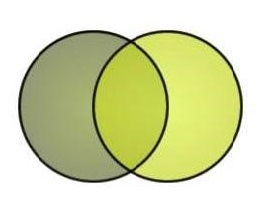# p-value

n=4

a: P-value ~
b: universal quantity

What:

"P-value is like an universal quantity for all the test statistics. Even we have different test statistics for different hypothesis using different data, the p-value between all of them are comparable to each other since it is a measure of support for. H0. Thus, this allows us to compare p-values while doing multiple test which is why "

Useful?
Writer: Yen-Chi Chen and Yu-Xiang Wang
LCC:
Where:
Date: May 5 2018 10:00 AM

a: P-value ~
b: an estimation of the probability

What:

"The P-value is like an estimation of the probability that the null hypothesis
is true. Because our objective it to reject the null hypothesis (i.e., to
disprove the null hypothesis), it is clear that small P-value are desired."

Useful?
Writer: Not Stated
LCC:
Where:
Date: May 5 2018 10:08 AM

a: P-value ~
b: a skyscraper above the lower level

What:

"Manhattan Plot is a scatter plot with the Y axis for the negative logarithm form of the p-values for each single nucleotide polymorphism (SNP), and the X axis for the genomic positions in each chromosome. The lower the p-value, the higher the negative logarithm of the p-value. A relatively extreme low p-value is like a skyscraper above the lower level 'buildings'. The SNPs can be classified in
to 22 chromosomes."

Useful?
Writer: Huei-Ling Chen, Jialin Xu
LCC:
Where:
Date: May 5 2018 10:12 AM

a: P-value ~
b: measurement of chance

What:

"In statistics the p-value is like a measurement of chance and the higher the p-value the higher the results are due to chance, and not due to the treatment."

Useful?
Writer: Malcolm Shane SIM
LCC:
Where:
Date: May 5 2018 10:14 AMMETAMIA is a free database of analogy and metaphor. Anyone can contribute or search. The subject matter can be anything. Science is popular, but poetry is encouraged. The goal is to integrate our fluid muses with the stark literalism of a relational database. Metamia is like a girdle for your muses, a cognitive girdle.